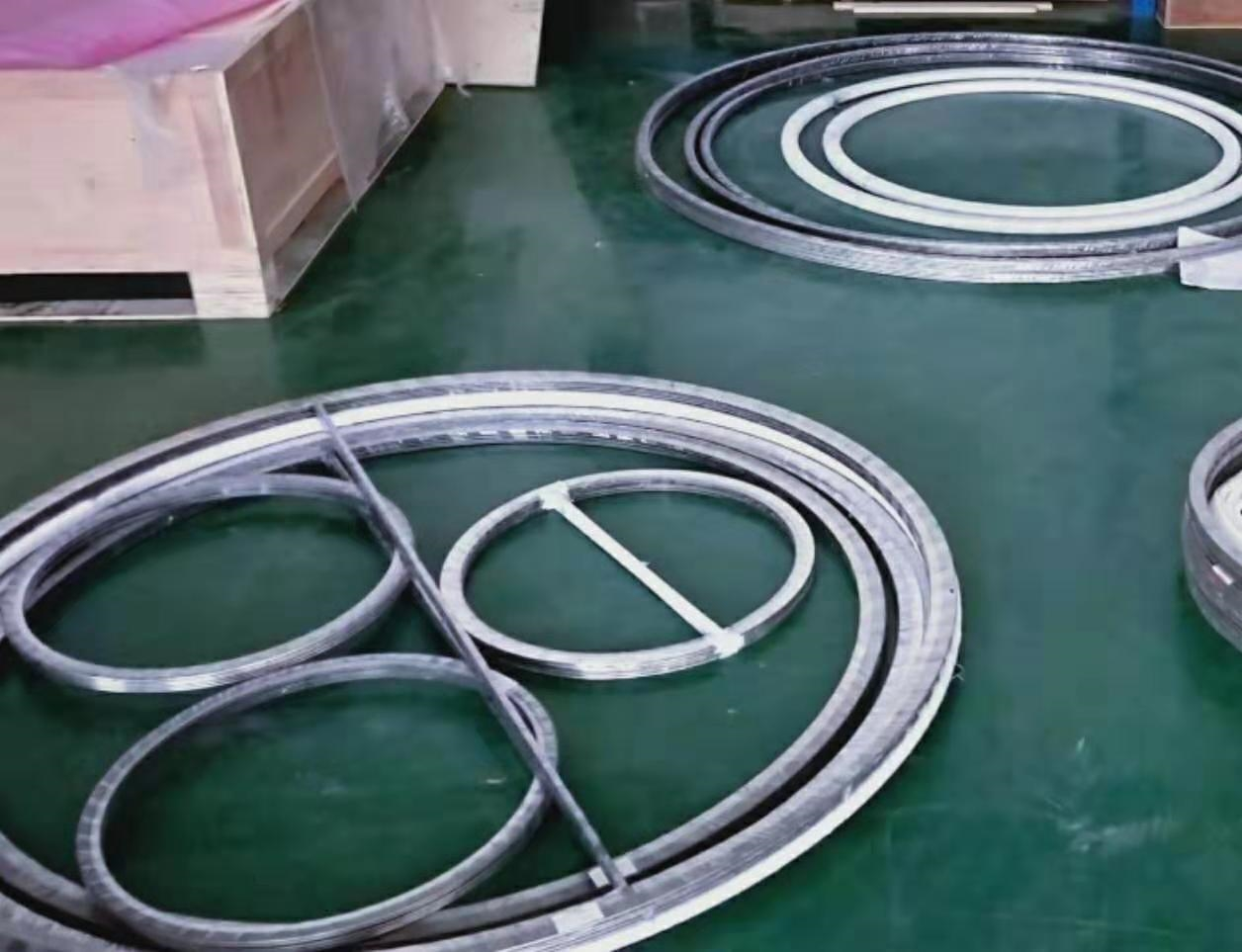# 机械阀门金属缠绕垫片

 价格 2
• 型号 DN15-DN2500
• 品牌
• 厂商性质 生产商
• 所在地 廊坊市

## 详细介绍

机械阀门金属缠绕垫片DIN (mm) ANSI B16.5 BS1560 (mm)Mss sp-44 API 605 公差 内环内径垫片内径 <=600 <=24" >=26" and <=34" ±0.5 >600 and <=800 >24" and <=34" >=36" and <=60" ±0.9 >800 and <=1600 >34" and <=60" ±1.3 >1600 >60" ±1.6 垫片外径 <=600 <=24" <=24" ±0.9 >600 and <=16000 >24" and <=60" >24" and <=60" ±1.8 1600 >60" ±2.2 外环外径 >=1/2" and <=60" ±0.9 <=600 <=24" 0-1.0 >600 and <=1000 >24" and <=34" 0-1.8 >1000 and <=1600" >34" and <=60 0-2.2## 其他推荐产品

### 提示15233666334